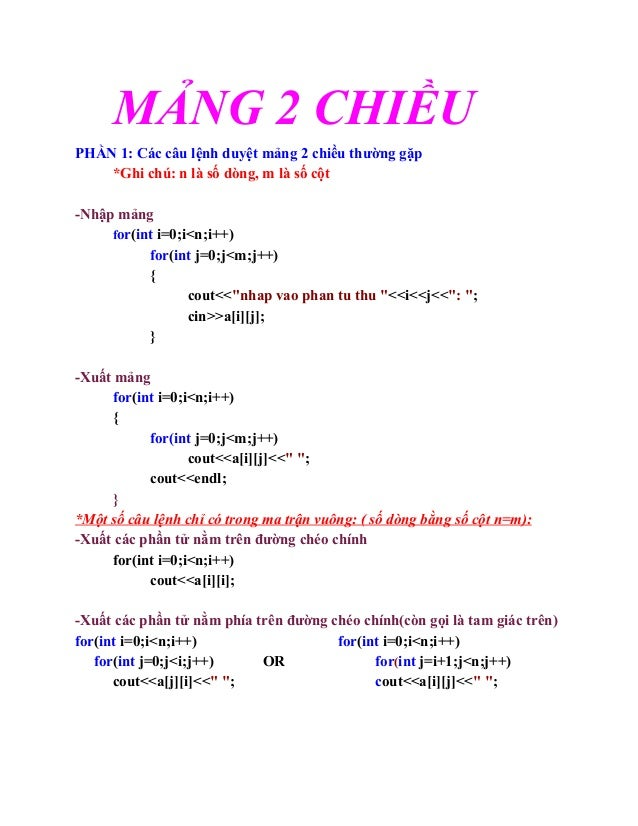Successfully reported this slideshow.
We use your LinkedIn profile and activity data to personalize ads and to show you more relevant ads. You can change your ad preferences anytime.Upcoming SlideShare
Loading in …5
×

# Mảng 2 chiều

tìm hiểu sâu về mảng 2 chiều, một thành phần quan trong nhất trong C và C++

• Full Name
Comment goes here.

Are you sure you want to Yes No
Your message goes here• Login to see the comments

### Mảng 2 chiều

1. 1. MẢNG 2 CHIỀU PHẦN 1: Các câu lệnh duyệt mảng 2 chiều thường gặp *Ghi chú: n là số dòng, m là số cột ­Nhập mảng for(int i=0;i<n;i++) for(int j=0;j<m;j++) { cout<<"nhap vao phan tu thu "<<i<<j<<": "; cin>>a[i][j]; } ­Xuất mảng for(int i=0;i<n;i++) { for(int j=0;j<m;j++) cout<<a[i][j]<<" "; cout<<endl; } *Một số câu lệnh chỉ có trong ma trận vuông: ( số dòng bằng số cột n=m): ­Xuất các phần tử nằm trên đường chéo chính for(int i=0;i<n;i++) cout<<a[i][i]; ­Xuất các phần tử nằm phía trên đường chéo chính(còn gọi là tam giác trên) for(int i=0;i<n;i++) for(int i=0;i<n;i++)      for(int j=0;j<i;j++) OR for(int j=i+1;j<n;j++) cout<<a[j][i]<<" "; cout<<a[i][j]<<" ";
2. 2. ­Xuất các phần tử nằm phía dưới đường chéo chính( còn gọi là tam giác dưới) for(int i=0;i<n;i++)       for(int j=0;j<i;j++) cout<<a[i][j]<<" "; ­Xuất các phần tử nằm trên đường chéo phụ for(int i=0;i<n;i++) cout<<a[i][n­1­i)<<” “; ­Xuất các phần tử nằm phía trên đường chéo phụ for(int i=0;i<n;i++) for(int i=0;i<n;i++)     for(int j=0;j<n­1­i;j++) OR          for(int j=n­i;j<n;j++) cout<<a[i][j]<<" ";                                         cout<<a[n­1­j][n­1­i]<<" "; ­Xuất các phần tử nằm phía dưới đường chéo phụ for(int i=0;i<n;i++)    for(int j=n­i;j<n;j++) cout<<a[i][j]<<" "; PHẦN 2: Một số bài tập tham tham khảo 1. viết hàm tính tổng các phần tử trên cùng một dòng. void tongpttrendong(int a[],int n,int m) { for(int i=0;i<n;++i) { int S=0; for(int j=0;j<m;++j) S+=a[i][j]; cout<<"dong "<<i<<" la: "<<S<<endl;
3. 3. } } 2. viết hàm tính tổng các phần tử trên cùng một cột void tongpttrencot(int a[],int n,int m) {   for(int i=0;i<m;i++)   { int s=0; for(int j=0;j<n;j++)  s=s+a[j][i]; cout<<"cot "<<i<<" la: "<<s<<endl;  } } 3. viết hàm tính tổng các phần tử thuộc đường chéo chính trong ma trận vuông.     int tongptdcchinh(int a[],int n,int m) {    int s=0;    for(int i=0;i<n;i++)  s=s+a[i][i];   return s; } 4.  Viết hàm tính tổng các giá trị lớn nhất trên mỗi dòng. int maxdong(int a[],int n,int m) {
4. 4.  int s=0; for(int i=0;i<n;i++)  {        int max=a[i];        for(int j=1;j<m;j++)                                  if(a[i][j]>max)                                       max=a[i][j];        s=s+max;                      }         return s; } 5.  Viết hàm tính giá trị trung bình của các phần tử nhỏ nhất trên mỗi cột. float tbmincot(int a[],int n,int m) { float s=0; for(int i=0;i<m;i++)  {   int min=a[i];   for(int j=1;j<n;j++)   if(a[j][i]<min)   min=a[j][i];   s=s+min; } return (float)s/m; }
5. 5. 6.  Viết hàm tính tổng các giá trị nhỏ nhất thuộc từng đường chéo song song với đường chéo chính chính. int tongminssdcchinh(int a[],int n,int m) { int s=0; for(int i=1;i<n;i++) { int m1=32768,m2=32768; for(int j=i;j<n;j++) { if(a[j][j­i]<m1) m1=a[j][j­i]; if(a[j­i][j]<m2) m2=a[j­i][j]; }  s=s+m1+m2;  } return s; } 7. Viết hàm tìm vị trí phần tử lớn nhất trong ma trận số nguyên void vtphantumax(int a[],int n,int m) { int d=0,c=0; for(int i=0;i<n;i++) for(int j=1;j<m;j++) if(a[d][c]<a[i][j]) { d=i; c=j; } cout<<"dong thu "<<d<<" cot thu "<<c;
6. 6. } 8. Viết hàm tìm vị trí phần tử chẳn cuối cùng trong mảng void vtchancuoicung(int a[],int n,int m) { int i,j; for( i=n­1;i>=0;i­­) { for(j=m­1;j>=0;j­­) if(a[i][j]%2==0) break; break; } cout<<"dong thu "<<i<<" ,cot thu "<<j; } 9. Viết hàm tìm phần tử âm lẻ và lớn nhất trong ma trận int ptamle(int a[],int n,int m) { for(int i=0;i<n;i++) for(int j=0;j<m;j++) if((a[i][j])<0 && (a[i][j])%2==­1) return a[i][j]; return 0; } int ptamlemax(int a[],int n,int m) { int max=ptamle(a,n,n); if(max!=0) { for(int i=0;i<n;i++) for(int j=0;j<m;j++) if(a[i][j]>max && a[i][j]<0 && a[i][j]%2==­1 )
7. 7. max=a[i][j]; } return max; } 10. Viết hàm tìm phần tử chẳn dương và nhỏ nhất trong ma trận int ptchanduong(int a[],int n,int m) { for(int i=0;i<n;i++) for(int j=0;j<m;j++) if((a[i][j])>0 && (a[i][j])%2==0) return a[i][j]; return 0; } int ptchanduongmin(int a[],int n,int m) { int min=ptchanduong(a,n,m); if(min!=0) { for(int i=0;i<n;i++) for(int j=0;j<m;j++) if(a[i][j]<min && a[i][j]>0 && a[i][j]%2==0 ) min=a[i][j]; } return min; } 11. Viết hàm tìm phần tử lớn nhất trên đường chéo chính của ma trận vuông int maxdcchinh( int a[],int n)      { int max=a;
8. 8. for(int i=1;i<n;i++)     if(a[i][i]>max) max=a[i][i]; return max;       } 12. Viết hàm hoán vị 2 dòng, hoán vị 2 cột trong ma trận void hoanvi(int &a,int &b) { int t=a; a=b; b=t; } void hoanvicot(int a[],int n,int m,int c1,int c2) { if((c1>=0 && c1<m)&&(c2>=0 && c2<m)) {      for(int i=0;i<n;i++) hoanvi(a[i][c1],a[i][c2]); } xuat(a,n,m); } void hoanvidong(int a[],int n,int m,int d1,int d2) { if((d1>=0 &&d1<n) &&(d2>=0 && d2<n))     { for(int j=0;j<m;j++) hoanvi(a[d1][j],a[d2][j]);    }       xuat(a,n,m); } 13. Viết hàm xóa một dòng, xóa một cột trong ma trận void xoacot(int a[],int n,int &m,int c)
9. 9. { if(c>=0 && c<m) { for(int i=0;i<n;i++) for(int j=c;j<m­1;j++) a[i][j]=a[i][j+1]; m­­; } xuat(a,n,m); } void xoadong(int a[],int &n,int m,int d) { if(d>=0 &&d<n) { for(int i=d;i<n­1;i++) for(int j=0;j<m;j++) a[i][j]=a[i+1][j]; n­­; } } 14. Viết hàm chèn  một dòng, chèn một cột trong ma trận void chencot(int a[],int n,int &m,int c) { if(c>=0 && c<m) { for(int i=0;i<n;i++) for(int j=m;j>c;j­­) a[i][j]=a[i][j­1]; m++; for(int i=0;i<n;i++) { cout<<"nhap phan tu dong "<<i<<" ";
10. 10. cin>>a[i][c]; } } } void chendong(int a[],int &n,int m,int d) { if(d>=0 &&d<n) { for(int i=n;i>d;i­­) for(int j=0;j<m;j++) a[i][j]=a[i­1][j]; n++; for(int j=0;j<m;j++) { cout<<"nhap phan tu cot "<<j<<" "; cin>>a[d][j]; } } } 15. Viết hàm tìm phần tử chẳn dương nhỏ nhất trong ma trận(Câu 10) int ChanDuongMin(int a[][Max],int d, int c) { int min=­1, i, j; for(i=0;i<d;i++) for(j=0;j<c;j++) if(a[i][j]%2==0 && a[i][j]>0) { if(min==­1) min=a[i][j]; else if(a[i][j]<min) min=a[i][j];
11. 11. } return min; } 16 .Viết hàm tìm vị trí xuất hiện đầu tiên của x trong ma trận void timx(float a[],int d,int c,float x,int &xd,int &xc) { xd=xc=­1; for(int i=0;i<d;i++) for(int j=0;j<c;j++) if(a[i][j]==x) { xd=i; xc=j; return; } 17 .Viết hàm xóa dòng có tổng lớn nhất của ma trận số thực(Trường hợp chỉ có một dòng có tổng lớn nhất) void xoadongtonglonnhat(float a[],int &d,int c) { float max,tong; int dong; tong=0; for(int j=0;j<c;j++) tong+=a[j]; max=tong; dong=0; for(int i=1;i<d;i++) { tong =0; for(int j=0;j<c;j++) tong+=a[i][j]; if(tong>max) { max=tong;
12. 12. dong=i; } } for(int i=dong;i<d;i++) { for(int j=0;j<c;j++) a[i][j]=a[i+1][j]; d­­; } } 18 .Viết hàm sắp xếp ma trận tăng dần từ trái sang phải từ trên xuống dưới void Interchangersort(int a[][Max],int d,int c) { for(int i=0;i<c*d­1;i++) for(int j=i+1;j<c*d;j++) if(a[i/c][i%c]>a[j/c][j%c]) hoanvi(a[i/c][i%c],a[j/c][j%c]); } design by admin void SapXep(int a[][Max],int d,int c) { int vtd,vtc,i,j; for(i=0;i<c*d­1;i++){ vtd=i/c;vtc=i%c; for(j=i+1;j<c*d;j++) if(a[vtd][vtc]>a[j/c][j%c]) {
13. 13. vtd=j/c; vtc=j%c; } Swap(a[i/c][i%c],a[vtd][vtc]); } } design by Trần văn cử *HÀM KHỞI TẠO MẢNG 2 CHIỀU Mảng Tăng:    void nhap(int a[][max],int d,int c)          {                 srand((unsigned) time(NULL));                 int t=abs(rand()%100);                 for(int i=0;i<d;i++)                       for(int j=0;i<c;j++)                          {                            a[i][j]=t+abs(rand()%100);                             t= a[i][j]; }               } Mảng Giảm:    void nhap(int a[][max],int d,int c)          {                 srand((unsigned) time(NULL));                 int t=abs(rand()%100);                 for(int i=d­1;i>=0;i­­)                       for(int j=c­1;i>=0;j­­)                          {                            a[i][j]=t+abs(rand()%100);                             t= a[i][j];
14. 14. }               } 19.Viết hàm tính tích 2 ma trận void Output_Matrix(int a[], int d, int c) { for(int i=0; i<d; i++) { for(int j=0; j<c; j++)             cout<<a[i][j]<<"t"; cout<<endl<<endl; } } // A(m,n) x B(n,k) = C(m,k) // A(da, ca) x B(db,cb) = C(da,cb) void Tich_Matrix(int a[], int b[], int ab[], int da, int ca, int db, int cb) { int i, j, k; if(ca != db) cout<<"Hai ma tran khong thoa tinh chat NHAN MA TRAN!"; else { cout<<"nnTich cua 2 Ma tran la: nn"; for(i=0; i<da; i++) for(j=0; j<cb; j++) ab[i][j]=0; for(i=0; i<da; i++)
15. 15. for(j=0; j<cb; j++) for(k=0; k<ca; k++) ab[i][j]+=a[i][k]*b[k][j]; Output_Matrix(ab, da, cb); } } 20.Viết hàm tính tổng 2 ma trận void Output_Matrix(int a[], int d, int c) { for(int i=0; i<d; i++) { for(int j=0; j<c; j++)             cout<<a[i][j]<<"t"; cout<<endl<<endl; } } void Sum_Matrix(int a[], int b[], int ab[], int da, int ca, int db, int cb) { if(da != db || ca != cb) cout<<"Hai ma tran khong thoa tinh chat cua CONG MA TRAN!"; else { cout<<"nnTong cua 2 Ma tran la: nn"; for(int i=0; i<da; i++)             for(int j=0; j<ca; j++) ab[i][j]=a[i][j]+b[i][j]; Output_Matrix(ab, da, ca); } } 20.Tính tổng các phần tử trên biên ma trận long tong_bien(int a[],int d,int c)
16. 16. { long tong=0; for(int i=0;i<c;i++) { tong += a[i]; tong += a[d­1][i]; } for(int i=1;i<d­1;i++) { tong += a[i]; tong += a[i][c­1]; } return tong; } 21.Tìm phần tử có giá trị lớn nhất trên biên ma trận long max_bien(int a[],int d,int c) { int max=a; for(int i=0;i<c;i++) { if(max<a[i]) max=a[i]; if(max<a[d­1][i])      max= a[d­1][i]; } for(int i=1;i<d­1;i++) { if(max<a[i]) max=a[i]; if(max<a[i][c­1]) max<a[i][c­1]; } return max;
17. 17. } 21.Ma trận ZigZag ngang #include <iostream> using namespace std; using namespace std; int a,d,c; void XuLy(int a[],int d,int c) {      int dem=1;      for(int i=0;i<d;i++)      {              if(i%2==0)              for(int j=0;j<c;j++)                a[i][j]=dem++;              else              for(int j=c­1;j>=0;j­­)                a[i][j]=dem++;      } } void Xuat(int a[],int d,int c) {      for(int i=0;i<d;i++)      {        for(int j=0;j<c;j++)        if(a[i][j]>=10)            cout<<a[i][j]<<" ";            else            cout<<" "<<a[i][j]<<" ";            cout<<endl<<endl;      } } void main() {
18. 18.     cout<<"Nhap dong: ";cin>>d;     cout<<"Nhap cot: ";cin>>c;     XuLy(a,d,c);     cout<<"Ma tran zigzag ngang la: nn";     Xuat(a,d,c); } 21.Ma trận xoắn ốc theo chiều kim đồng hồ #include <iostream> using namespace std; int a,d,c; void TaoMaTran() { int trai=0,tren=0,phai=c,duoi=d,dem=0,soluong=c*d; while(dem<soluong) { for(int i=trai;i<phai;i++) if(dem<soluong) a[tren][i]=dem++; tren++; for(int i=tren;i<duoi;i++) if(dem<soluong) a[i][phai­1]=dem++; phai­­; for(int i=phai­1;i>=trai;i­­) if(dem<soluong) a[duoi­1][i]=dem++; duoi­­; for(int i=duoi­1;i>=tren;i­­) if(dem<soluong) a[i][trai]=dem++; trai++; }
19. 19. } void XuatMaTran() { for(int i=0;i<d;i++) { for(int j=0;j<c;j++) if(a[i][j]>=10) cout<<a[i][j]<<" "; else cout<<" "<<a[i][j]<<" "; cout<<endl<<endl; } } void  main() { cout<<"Nhap dong,cot: "; cin>>d>>c; TaoMaTran(); cout<<"Ma tran xoan oc la: n"; XuatMaTran(); }